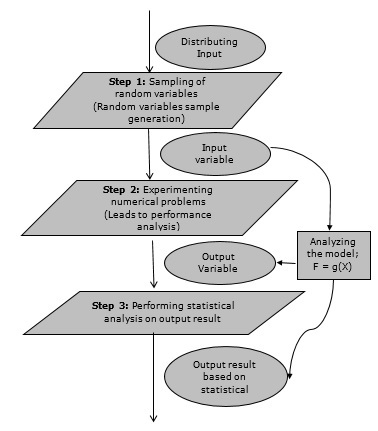# Monte Carlo Simulation

#### Creo Parametric-3D Modelling Software (Professional)

130 Lectures 16.5 hours

#### Learn Product Design and Modelling with Photoshop 3D

11 Lectures 53 mins

#### Hydraulic System Modelling with Matlab and System Dynamics

10 Lectures 2.5 hours

Monte Carlo simulation is a computerized mathematical technique to generate random sample data based on some known distribution for numerical experiments. This method is applied to risk quantitative analysis and decision making problems. This method is used by the professionals of various profiles such as finance, project management, energy, manufacturing, engineering, research & development, insurance, oil & gas, transportation, etc.

This method was first used by scientists working on the atom bomb in 1940. This method can be used in those situations where we need to make an estimate and uncertain decisions such as weather forecast predictions.

## Monte Carlo Simulation ─ Important Characteristics

Following are the three important characteristics of Monte-Carlo method −

• Its output must generate random samples.
• Its input distribution must be known.
• Its result must be known while performing an experiment.

## Monte Carlo Simulation ─ Advantages

• Easy to implement.
• Provides statistical sampling for numerical experiments using the computer.
• Provides approximate solution to mathematical problems.
• Can be used for both stochastic and deterministic problems.

## Monte Carlo Simulation ─ Disadvantages

• Time consuming as there is a need to generate large number of sampling to get the desired output.

• The results of this method are only the approximation of true values, not the exact.

## Monte Carlo Simulation Method ─ Flow Diagram

The following illustration shows a generalized flowchart of Monte Carlo simulation.